• Shuffle
Toggle On
Toggle Off
• Alphabetize
Toggle On
Toggle Off
• Front First
Toggle On
Toggle Off
• Both Sides
Toggle On
Toggle Off
Toggle On
Toggle Off
Front

## Card Range To Study

throughPlay buttonPlay buttonProgress

1/26

Click to flip

Use LEFT and RIGHT arrow keys to navigate between flashcards;

Use UP and DOWN arrow keys to flip the card;

H to show hint;

 molarity-M mass percent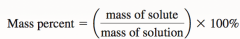-aka weight percent mole fraction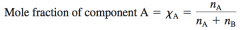-X molality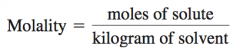-m normality -N-number of equivalents per liter of solution-acid-base, divide by the number of H+ ions -redox, divide by number of e- enthalpy (heat) of solution (delta H soln)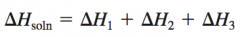-may be positive (energy absorbed, endo)-may be negative (energy released, exo)-steps 1 and 2 are usually endo, step 3 is usually eco formation of liquid solution enthalpy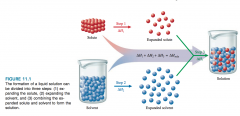energy and the formation of solutions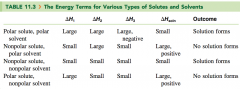-smaller enthalpies have a higher chance of forming solutions Henry's law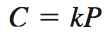-amount of a gas dissolved in a solution is directly proportional to the pressure of the gas above the solution. -C: concentration of dissolved gas-k: constant characteristic of a particular solution-P: partial pressure of gaseous solute above the solution Raoult's Law, non volatile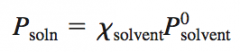-Psoln is the observed vapor pressure of the soln-Xsolv is the mole fraction of the solvent-P0solv is the vapor pressure of the pure solvent Raoult's Law, volatile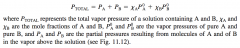raoult's law deviations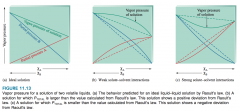-negative deviation: lower vapor pressure than predicted, strong interactions-positive deviation: higher vapor pressure than predicted, weak interactions raoult's law deviations and relation to enthalpy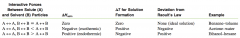-negative: large amounts of energy released due to strong interactions-positive: small amounts of energy released due to weak interactions boiling point elevation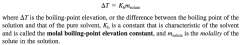a nonvolatile solute elevates the boiling point of the solvent common k constants for boiling and freezing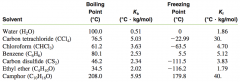freezing point depression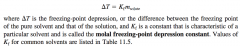osmotic pressure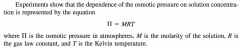isotonic solution solutions that have identical osmotic pressures van't hoff factor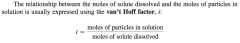-The expected value for i can be calculated for a salt by noting the number of ions per formula unit. For example, for NaCl, i is 2; for K2SO4, i is 3; and for Fe3(PO4)2, i is 5. -van't hoff factor is used for salts, not non electrolytes-weak acids only don't have it Expected and observed van't hoff factors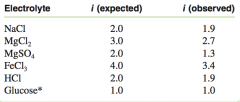-van't hoff factor is used for salts, glucose is a non electrolyte Enthalpy calculation for solutions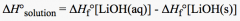aq - solid-negative is exo, solubility decreases as temp rises-positive is endo, solubility increases as temp rises cubic centimeter equivalent to a mL solubility -ionic compounds dissolve readily in water-the longer the hydrocarbon chain, the more non polar it is-water is polar hydration in an aqueous solution -Interactions between the water molecules and ions are stronger with smaller ions.-Interactions between the water molecules and ions increases as the charge on the ions increase. how to find empirical formulas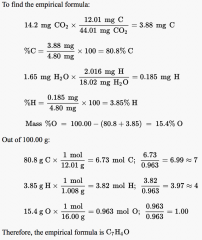1. find the percent of the total of each atom2. multiply by 100g3. multiply by the molecular weight of the atom4. round to the nearest integer how to find molecular formulas 1. compare molecular weight of the empirical formula against a determined molecular weight2. multiply the empirical formula by the multiple difference